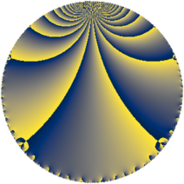# Properties

 Label 72.3.hLevel $72$ Weight $3$ Character orbit 72.h Rep. character $\chi_{72}(53,\cdot)$ Character field $\Q$ Dimension $8$ Newform subspaces $1$ Sturm bound $36$ Trace bound $0$

# Related objects

## Defining parameters

 Level: $$N$$ $$=$$ $$72 = 2^{3} \cdot 3^{2}$$ Weight: $$k$$ $$=$$ $$3$$ Character orbit: $$[\chi]$$ $$=$$ 72.h (of order $$2$$ and degree $$1$$) Character conductor: $$\operatorname{cond}(\chi)$$ $$=$$ $$24$$ Character field: $$\Q$$ Newform subspaces: $$1$$ Sturm bound: $$36$$ Trace bound: $$0$$

## Dimensions

The following table gives the dimensions of various subspaces of $$M_{3}(72, [\chi])$$.

Total New Old
Modular forms 28 8 20
Cusp forms 20 8 12
Eisenstein series 8 0 8

## Trace form

 $$8q + 4q^{4} + O(q^{10})$$ $$8q + 4q^{4} + 28q^{10} - 72q^{16} - 88q^{22} + 40q^{25} + 104q^{28} - 128q^{31} + 212q^{34} - 240q^{40} - 136q^{46} + 24q^{49} + 248q^{52} + 256q^{55} + 260q^{58} - 32q^{64} - 312q^{70} - 160q^{73} + 304q^{76} - 384q^{79} - 188q^{82} - 256q^{88} - 216q^{94} - 192q^{97} + O(q^{100})$$

## Decomposition of $$S_{3}^{\mathrm{new}}(72, [\chi])$$ into newform subspaces

Label Dim. $$A$$ Field CM Traces $q$-expansion
$$a_2$$ $$a_3$$ $$a_5$$ $$a_7$$
72.3.h.a $$8$$ $$1.962$$ 8.0.$$\cdots$$.2 None $$0$$ $$0$$ $$0$$ $$0$$ $$q-\beta _{2}q^{2}+(1+\beta _{5})q^{4}+(-\beta _{2}-\beta _{4}+\cdots)q^{5}+\cdots$$

## Decomposition of $$S_{3}^{\mathrm{old}}(72, [\chi])$$ into lower level spaces

$$S_{3}^{\mathrm{old}}(72, [\chi]) \cong$$ $$S_{3}^{\mathrm{new}}(24, [\chi])$$$$^{\oplus 2}$$